Plane

Chapter 11 Class 12 Three Dimensional Geometry
Serial order wise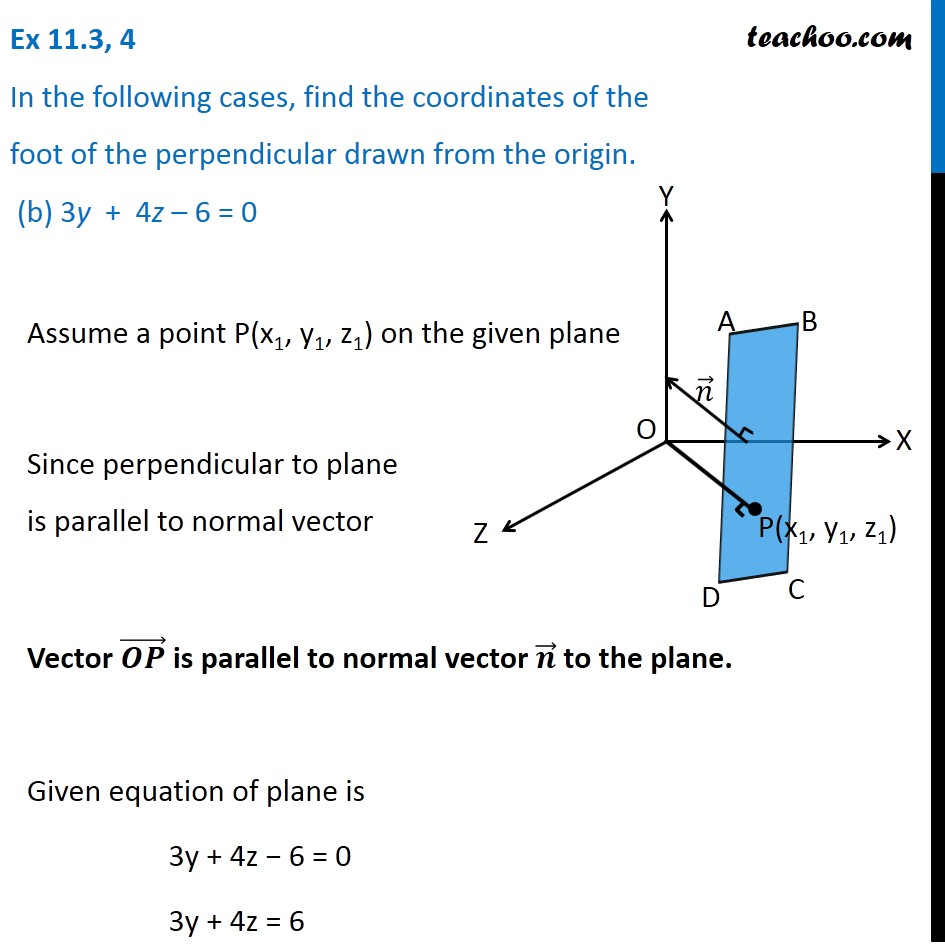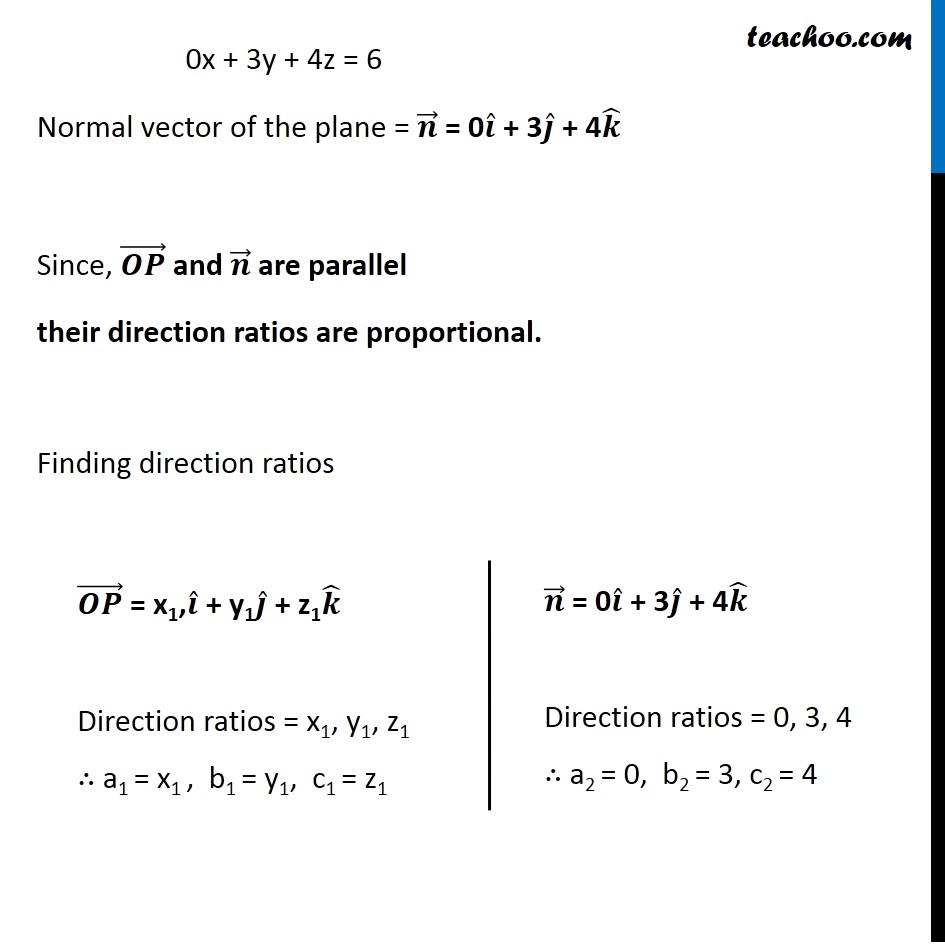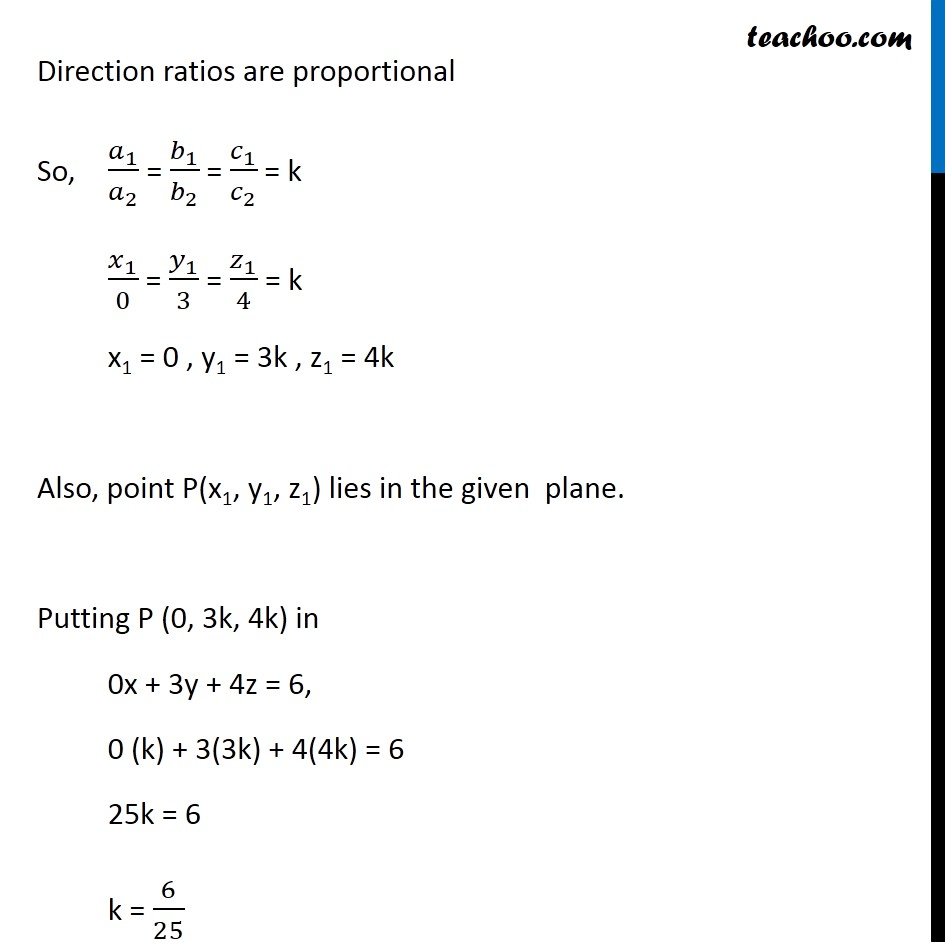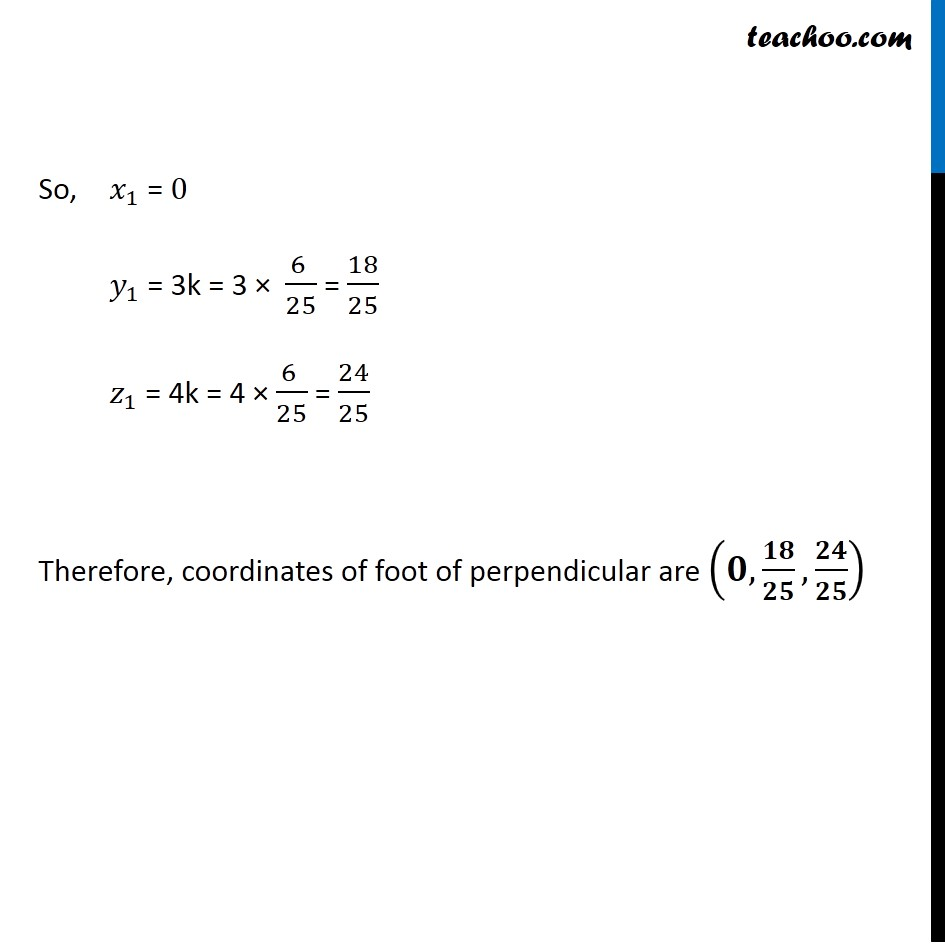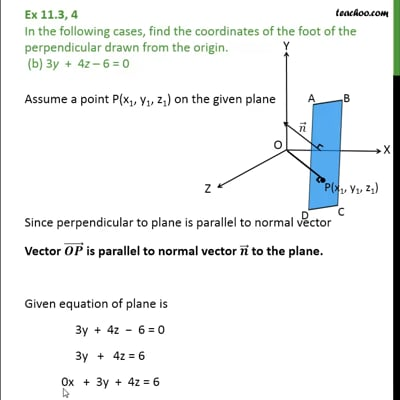This video is only available for Teachoo black users

Learn in your speed, with individual attention - Teachoo Maths 1-on-1 Class

### Transcript

Question 4 In the following cases, find the coordinates of the foot of the perpendicular drawn from the origin. (b) 3y + 4z 6 = 0 Assume a point P(x1, y1, z1) on the given plane Since perpendicular to plane is parallel to normal vector Vector is parallel to normal vector to the plane. Given equation of plane is 3y + 4z 6 = 0 3y + 4z = 6 0x + 3y + 4z = 6 Since, and are parallel their direction ratios are proportional. Finding direction ratios Direction ratios are proportional So, 1 2 = 1 2 = 1 2 = k 1 0 = 1 3 = 1 4 = k x1 = 0 , y1 = 3k , z1 = 4k Also, point P(x1, y1, z1) lies in the given plane. Putting P (0, 3k, 4k) in 0x + 3y + 4z = 6, 0 (k) + 3(3k) + 4(4k) = 6 25k = 6 k = 6 25 So, 1 = 0 1 = 3k = 3 6 25 = 18 25 1 = 4k = 4 6 25 = 24 25 Therefore, coordinates of foot of perpendicular are ,